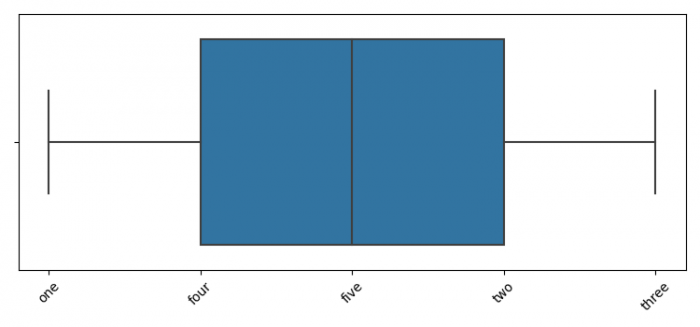# How to create a Boxplot with Matplotlib?

To create a Boxplot with Matplotlib, we can take the following steps −

• Set the figure size and adjust the padding between and around the subplots.

• Make a list of xticks.

• Plot a boxplot with xticks data.

• Set xticks and xtick labels with 45° rotation.

• To display the figure, use show() method.

## Example

import seaborn as sns
from matplotlib import pyplot as plt

plt.rcParams["figure.figsize"] = [7.50, 3.50]
plt.rcParams["figure.autolayout"] = True

xticks = [1, 4, 5, 2, 3]
ax = sns.boxplot(xticks)

ax.set_xticks(xticks)
ax.set_xticklabels(["one", "two", "three", "four", "five"], rotation=45)

plt.show()

## Output